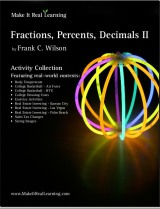^
You are here: HomeMake It Real Learning → Fractions, Decimals, Percents II

# Make It Real Learning Fractions, Decimals, Percents II - real-life math workbook46 pages
(10 activities)

## Introduction

The Make It Real Learning Fractions, Decimals, Percents II workbook focuses on real-world situations that may be effectively analyzed using decimals, ratios, histograms, and percents. This book suits best middle and high school, meaning that some activities here suit best middle school students, and some suit best high school students.

From determining how to analyze a real estate deal to determining college housing costs, learners get to use mathematics in meaningful ways. Each activity integrates real world information, companies, and issues (e.g. BYU, Air Force, college basketball, real estate investing, sizing images, sales tax), not just "realistic" data.

There are multiple ways to use the activities of the book in a teaching environment. The activities are an excellent tool for stimulating mathematical discussions in a small group setting. Due to the challenging nature of each activity, group members are motivated to brainstorm problem solving strategies together. The interesting real world contexts motivate them to want to solve the problems. The activities may also be used for individual projects and class-wide discussions.

As a ready-resource for teachers, the workbook also includes completely worked-out solutions for each activity. To make it easier for teachers to assess student work, the solutions are included on a duplicate copy of each activity.

The mathematical objectives of the activities fall within the standards of the National Council of Teachers of Mathematics.

## Fractions, Decimals, Percents II Activity List

Activity TitleMathematical Objectives
Body Temperature:
Working with Histograms
Create a frequency table
Create a histogram from a frequency table
Calculate percents
Interpret the meaning of histograms
Working with Histograms
Create a frequency table
Create a histogram from a frequency table
Calculate percents
Interpret the meaning of histograms
College Housing Costs:
Using Ratios
Create fractions from a verbal description
Simplify fractions
Find the indicated fraction of a number
Convert decimal numbers to percents
Exercise Activities:
Working with Histograms
Create a frequency table and histogram
Calculate percents
Interpret the meaning of histograms
Calculate an average
Real Estate Investing - Kansas City:
Using Ratios to Analyze Deals
Calculate ratios in a real world context
Interpret the meaning of ratios in a real world context
Convert a fraction to a percent
Real Estate Investing - Las Vegas:
Using Ratios to Analyze Deals
Calculate ratios in a real world context
Interpret the meaning of ratios in a real world context
Find the given percent of a number
Convert a fraction to a percent
Real Estate Investing - Palm Beach:
Using Ratios to Analyze Deals
Calculate ratios in a real world context
Interpret the meaning of ratios in a real world context
Convert a fraction to a percent
Sales Tax Changes:
Working with Percents
Convert a percent to a decimal
Create and solve a linear equation
Multiply two numbers
Sizing Images:
Using Ratios
Calculate the aspect ratio of an image
Solve equations involving ratios

Bundle specials!

Activity Library, Volume I. Price: \$39.99
Includes 11 Make It Real Learning activity workbooks for the price of 8! You get three workbooks for free!

Activity Library, Volume II. Price: \$39.99
Includes 11 Make It Real Learning activity workbooks for the price of 8! You get three workbooks for free!

States by the Numbers Bundle. Price: \$19.99
Inludes all 50 States by the Numbers workbooks at a whopping 87% discount!
(Normal price would be 50 × \$2.99 = \$149.50)

By purchasing any of the Make It Real Learning workbooks, permission IS granted for the teacher (or parent) to reproduce this material to be used with his/her students in a teaching situation; not for commercial resale. However, you are not permitted to share the material with another teacher.

In other words, you are permitted to make copies for the students/children you are teaching, but not for other teachers' usage.

WAIT!

Receive my monthly collection of math tips & resources directly in your inbox — and get a FREE Math Mammoth book!You can unsubscribe at any time.

### Math Mammoth Tour

Confused about the different options? Take a virtual email tour around Math Mammoth! You'll receive:

An initial email to download your GIFT of over 400 free worksheets and sample pages from my books. Six other "TOURSTOP" emails that explain the important things and commonly asked questions concerning Math Mammoth curriculum. (Find out the differences between all these different-colored series!)

This way, you'll have time to digest the information over one or two weeks, plus an opportunity to ask me personally about the curriculum.
A monthly collection of math teaching tips & Math Mammoth updates (unsubscribe any time)### "Mini" Math Teaching Course

This is a little "virtual" 2-week course, where you will receive emails on important topics on teaching math, including:

- How to help a student who is behind
- Troubles with word problems
- Teaching multiplication tables
- Why fractions are so difficult
- The value of mistakes
- Should you use timed tests
- And more!

A GIFT of over 400 free worksheets and sample pages from my books right in the very beginning.A monthly collection of math teaching tips & Math Mammoth updates (unsubscribe any time)
Enter your email to receive math teaching tips, resources, Math Mammoth news & sales, humor, and more! I tend to send out these tips about once monthly, near the beginning of the month, but occasionally you may hear from me twice per month (and sometimes less often).• A GIFT of over 400 free worksheets and sample pages from my books.